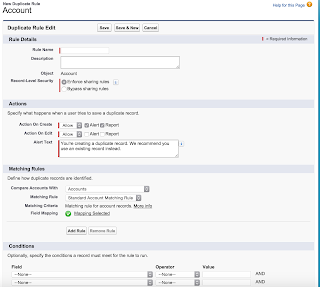## Thursday, July 30, 2015

### Making SalesForce More Welcoming

A major challenge with any SalesForce implementation is how to make it easier for users to use SalesForce!  Often users, when they first log in, can be confused by the SalesForce interface.  Or, even if they familiar the interface, the way that one organization is using SalesForce can be very different from another.

So, often administrators believe that more training is the solution for end users.  Which, is a noble solution.  However, with organizations using SalesForce in so many different ways, with so many different user groups, that can be a challenging solution.

An easy solution that can be customized for different user groups is to have an introduction focused page.   This page can include text, videos and more all in one place, right when the user logs in.  This makes it very obvious for the user where they can find help, but also get a quick overview.  And perhaps even better, is that this solution could be done in an afternoon to get at least get the base framework up.

I have seen these made, and made many myself, for organizations to help with user adoption.  We will also look soon at another tool you can have integrated on any page within SalesForce.Sample Introduction Focused Page

## Monday, July 27, 2015

### Two Useful Excel Formulas You Will Need

With SalesForce, ideally you can do everything up in the cloud.  However, there are times you have to prepare data for upload into SalesForce.  When that happens, often it is fairly easy to prepare data for import.  But if you are doing an update (on a record), because of the way SalesForce structures their record IDs, there are two major gotchas!  They are:
• The Record IDs are case sensitive.  So, 00Od0000004PuiV and 00Od0000004Puiv would take you to different records
• The Record IDs are sometimes 15 characters and sometimes 18 characters (which means when you update a record, you are updating the wrong record if you are not using the right ID)
So, as a result of this, there are some tricks/formulas you can use in Excel to make things easier.  Here they are:
• To lookup IDs, case sensitively, you can use this formula:
 {=INDEX(NEW!\$C\$2:\$C\$7050,MATCH(TRUE,EXACT(NEW!\$A\$2:\$A\$7050,A2),0))
For this formula, cells \$C\$2:\$C\$7050 would be where you want the results to be put in Excel.  Cells \$A\$2:\$A\$7050 would be where your current IDs start.  Then ensure you put it in as an Array (when editing the formula press Shift and Command and Enter at the same time)
• To look at a 15 character ID and convert it to an 18 character ID, you can use this formula:  =A2&LOOKUP(IF(AND(CODE(RIGHT(LEFT(\$A2,5),1))>=65,CODE(RIGHT(LEFT(\$A2,5),1))<=90),1,0) & IF(AND(CODE(RIGHT(LEFT(\$A2,4),1))>=65,CODE(RIGHT(LEFT(\$A2,4),1))<=90),1,0) & IF(AND(CODE(RIGHT(LEFT(\$A2,3),1))>=65,CODE(RIGHT(LEFT(\$A2,3),1))<=90),1,0)& IF(AND(CODE(RIGHT(LEFT(\$A2,2),1))>=65,CODE(RIGHT(LEFT(\$A2,2),1))<=90),1,0)& IF(AND(CODE(LEFT(\$A2,1))>=65,CODE(LEFT(\$A2,1))<=90),1,0),{"00000","00001","00010","00011","00100","00101","00110","00111","01000","01001","01010","01011","01100","01101","01110","01111","10000","10001","10010","10011","10100","10101","10110","10111","11000","11001","11010","11011","11100","11101","11110","11111"},{"A","B","C","D","E","F","G","H","I","J","K","L","M","N","O","P","Q","R","S","T","U","V","W","X","Y","Z","0","1","2","3","4","5"}) & LOOKUP(IF(AND(CODE(RIGHT(LEFT(\$A2,10),1))>=65,CODE(RIGHT(LEFT(\$A2,10),1))<=90),1,0) & IF(AND(CODE(RIGHT(LEFT(\$A2,9),1))>=65,CODE(RIGHT(LEFT(\$A2,9),1))<=90),1,0) & IF(AND(CODE(RIGHT(LEFT(\$A2,8),1))>=65,CODE(RIGHT(LEFT(\$A2,8),1))<=90),1,0)& IF(AND(CODE(RIGHT(LEFT(\$A2,7),1))>=65,CODE(RIGHT(LEFT(\$A2,7),1))<=90),1,0)& IF(AND(CODE(RIGHT(LEFT(\$A2,6),1))>=65,CODE(RIGHT(LEFT(\$A2,6),1))<=90),1,0),{"00000","00001","00010","00011","00100","00101","00110","00111","01000","01001","01010","01011","01100","01101","01110","01111","10000","10001","10010","10011","10100","10101","10110","10111","11000","11001","11010","11011","11100","11101","11110","11111"},{"A","B","C","D","E","F","G","H","I","J","K","L","M","N","O","P","Q","R","S","T","U","V","W","X","Y","Z","0","1","2","3","4","5"})&LOOKUP(IF(AND(CODE(RIGHT(LEFT(\$A2,15),1))>=65,CODE(RIGHT(LEFT(\$A2,15),1))<=90),1,0) & IF(AND(CODE(RIGHT(LEFT(\$A2,14),1))>=65,CODE(RIGHT(LEFT(\$A2,14),1))<=90),1,0) & IF(AND(CODE(RIGHT(LEFT(\$A2,13),1))>=65,CODE(RIGHT(LEFT(\$A2,13),1))<=90),1,0)& IF(AND(CODE(RIGHT(LEFT(\$A2,12),1))>=65,CODE(RIGHT(LEFT(\$A2,12),1))<=90),1,0)& IF(AND(CODE(RIGHT(LEFT(\$A2,11),1))>=65,CODE(RIGHT(LEFT(\$A2,11),1))<=90),1,0),{"00000","00001","00010","00011","00100","00101","00110","00111","01000","01001","01010","01011","01100","01101","01110","01111","10000","10001","10010","10011","10100","10101","10110","10111","11000","11001","11010","11011","11100","11101","11110","11111"},{"A","B","C","D","E","F","G","H","I","J","K","L","M","N","O","P","Q","R","S","T","U","V","W","X","Y","Z","0","1","2","3","4","5"})Sample of the Record ID Conversion Formula in Excel

## Thursday, July 9, 2015

### Having Partner Community Uses Use Outlook

As many of you have likely discovered, SalesForce's Outlook Plug-ins only work with Full SalesForce Licenses.  They are not available for Community users!

This is an issue if you have Community users that want to save emails.  You want to encourage that behaviour!  So, I recently found a solution that works!  There is a tool called LinkPoint 360.  With this tool, Community Users (for a few dollars a month) can have the ability to use Outlook and have access to saving emails into SalesForce!  This is a nice little solution without having to upgrade your community users to full SalesForce licenses.  I have detailed install instructions if you want them!

## Tuesday, July 7, 2015

### Managing Duplicates

A big challenge for a lot of Sales and Marketing team previously was managing duplicates in SalesForce.  That's because, prior to Spring 15, there was no way to handle this issue until the duplicates were already in the system.  Then, at that time you could merge them!  But what if you wanted to stop duplicates from happening?  Previously you had to look at third party solutions!  However that is no longer the case.  Now SalesForce has this functionality built in!

So, how does it work? Well, it requires you to create matching rules.  You can do this under Setup>Administration Setup>Data.com Administration>Duplicate Management>Matching Rules.  Within here you can set what fields should be looked at for matches.  For example, if you were doing leads, you would likely choose First Name, Last Name and Email.Screenshot: Matching Rules

Now, once you have setup your matching rules, you have to make your "Duplicate Rules".  You can do this under Setup>Administration Setup>Data.com Administration>Duplicate Management.>Duplicate Rules.  Within here, you are specifying when should you matching rule be checked (when records have this value in them) and what should display to the user then they try to create the duplicate.Screenshot: Duplicate Rules

Like most SalesForce implemented features where third party solutions previously existed, it is fairly well implemented and easy to use.